## Instantaneous Power Formula:

Instantaneous Power Formula – In a purely resistive circuit, all the energy delivered by the source is dissipated in the form of heat by the resistance. In a purely reactive (inductive or capacitive) circuit, all the energy delivered by the source is stored by the inductor or capacitor in its magnetic or electric field during a portion of the voltage cycle, and then is returned to the source during another portion of the cycle, so that no net energy is transferred. When there is complex impedance in a circuit, part of the energy is alternately stored and returned by the reactive part, and part of it is dissipated by the resistance. The amount of energy dissipated is determined by the relative values of resistance and reactance.

Consider a circuit having complex impedance. Let υ(t) = Vm cos ωt be the voltage applied to the circuit and let i(t) = Im cos (ωt + θ) be the corresponding current flowing through the circuit. Then the power at any instant of time is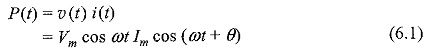From Eq. 6.1, we get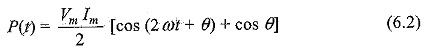Equation 6.2 represents Instantaneous Power Formula. It consists of two parts. One is a fixed part, and the other is time-varying which has a frequency twice that of the voltage or current waveforms: The voltage, current and power waveforms are shown in Figs 6.1 and 6.2.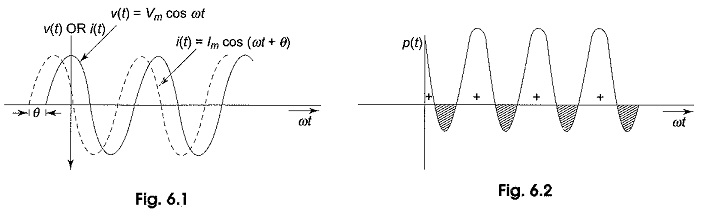Here, the negative portion (hatched) of the power cycle represents the power returned to the source. Figure 6.2 shows that the instantaneous power is negative whenever the voltage and current are of opposite sign. In Fig. 6.2, the positive portion of the power is greater than the negative portion of the power; hence the average power is always positive, which is almost equal to the constant part of the Instantaneous Power Formula (Eq. 6.2). The positive portion of the power cycle varies with the phase angle between the voltage and current waveforms. If the circuit is pure resistive, the phase angle between voltage and current is zero; then there is no negative cycle in the P(t) curve. Hence, all the power delivered by the source is completely dissipated in the resistance.

If θ becomes zero in Eq. 6.1, we get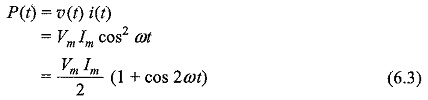The waveform for Eq. 6.3, is shown in Fig. 6.3, where the power wave has a frequency twice that of the voltage or current. Here the average value of power is VmIm/2.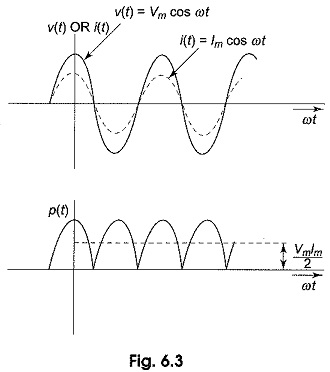When phase angle θ is increased, the negative portion of the power cycle increases and lesser power is dissipated.When θ becomes π/2, the positive and negative portions of the power cycle are equal. At this instant, the power dissipated in the circuit is zero, i.e. the power delivered to the load is returned to the source.

Scroll to Top